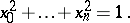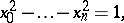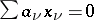# Difference between revisions of "Weierstrass coordinates"

Jump to: navigation, search

A type of coordinates in an elliptic space. Let \$M^n\$ be an elliptic space obtained by the identification of diametrically-opposite points of the unit sphere \$S^n\$ in \$(n+1)\$-dimensional Euclidean space. The Weierstrass coordinates \$(x_0 ,\ldots, x_n)\$ of a point in \$M^n\$ are the orthogonal Cartesian coordinates of the point of \$S^n\$ that corresponds to it. Since the isometric mapping of \$M^n\$ into \$S^n\$ is not single-valued, Weierstrass coordinates are defined up to sign. A hyperplane in \$M^n\$ is given by a homogeneous linear equationNamed after K. Weierstrass, who used these coordinates in his courses on Lobachevskii geometry in 1872.

#### Comments

These coordinates for elliptic space can be normalized so thatThe analogous Weierstrass coordinates for hyperbolic space satisfywith the same equationfor a hyperplane.

How to Cite This Entry:
Weierstrass coordinates. Encyclopedia of Mathematics. URL: http://encyclopediaofmath.org/index.php?title=Weierstrass_coordinates&oldid=26263
This article was adapted from an original article by E.V. Shikin (originator), which appeared in Encyclopedia of Mathematics - ISBN 1402006098. See original article